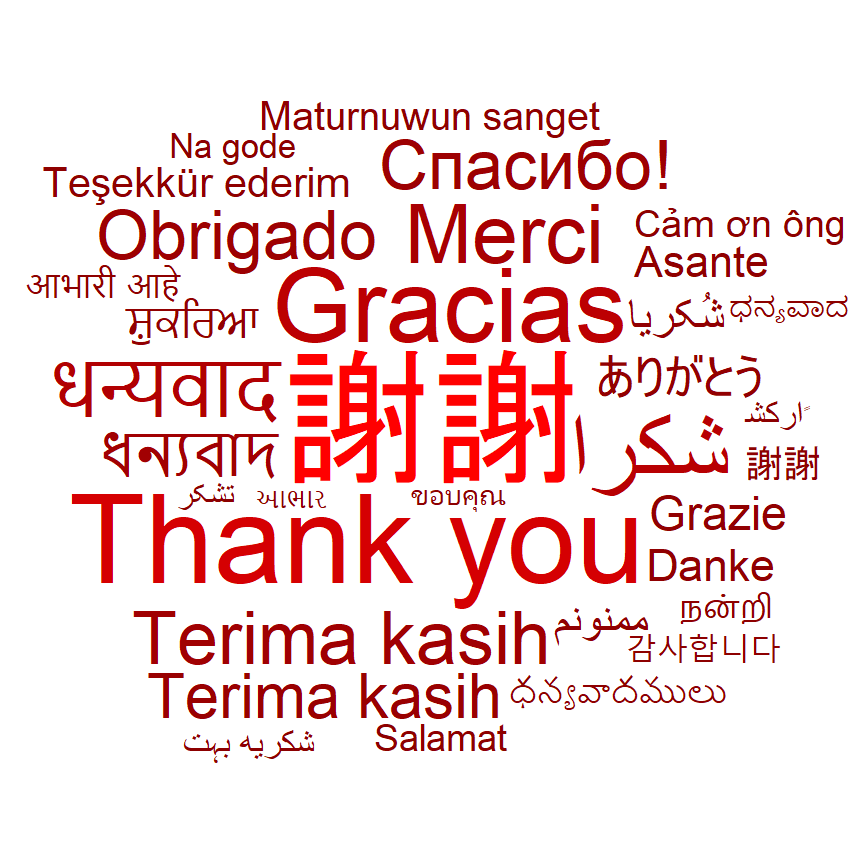# Word cloud in ggplot2 with ggwordcloud

Erwan Le Pennec

## Sample data

In order to create a word cloud with `ggwordcloud` you will need at least a data frame containing the words and optionally a numerical column which will be used to scale the texts. In this tutorial we are going to use the `thankyou_words_small` data set from the package for illustration purposes.

``````# install.packages("ggwordcloud")
library(ggwordcloud)

df <- thankyou_words_small``````

## Word cloud with `ggwordcloud`

Basic word cloud

`ggwordcloud` provides a ggplot2 geom named `geom_text_wordcloud` for creating word clouds. Use your data frame and pass the column containing the texts to the `label` argument of `aes` and use the `geom_text_wordcloud` function. Note that we are setting a seed to keep the example reproducible, as the algorithm used for placing the texts involves some randomness.

``````# install.packages("ggwordcloud")
library(ggwordcloud)

# Data
df <- thankyou_words_small

set.seed(1)
ggplot(df, aes(label = word)) +
geom_text_wordcloud() +
theme_minimal()``````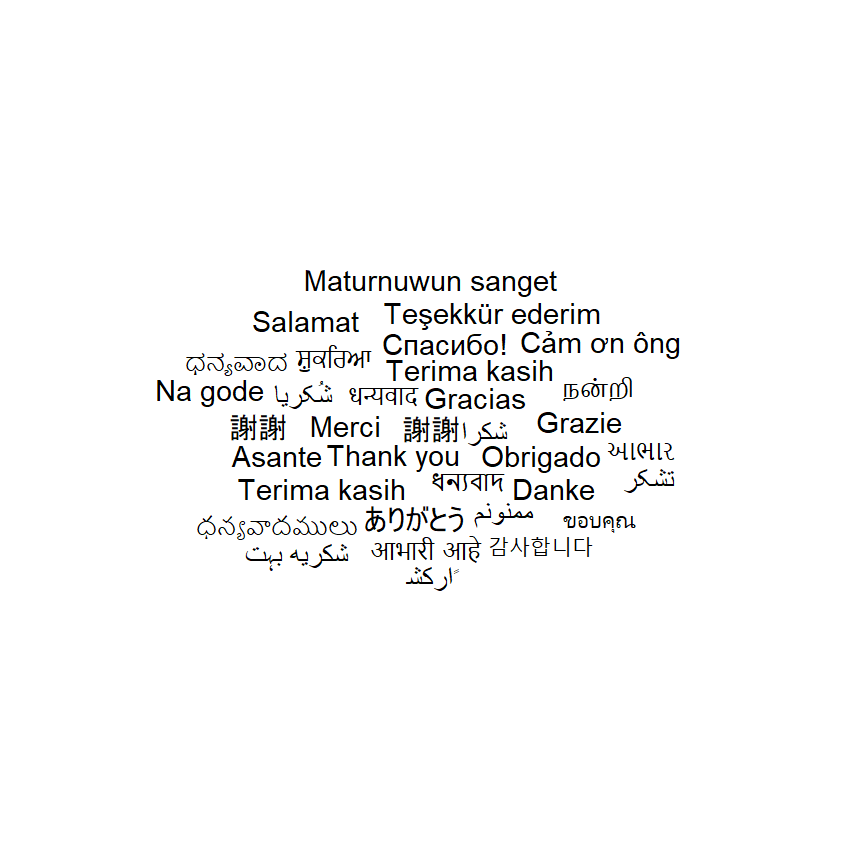Size of the text based on a variable

So far all the words had the same size. If you want to set the size based on a numerical variable you can pass it to the `size` argument of `aes`.

``````# install.packages("ggwordcloud")
library(ggwordcloud)

# Data
df <- thankyou_words_small

set.seed(1)
ggplot(df, aes(label = word, size = speakers)) +
geom_text_wordcloud() +
theme_minimal()``````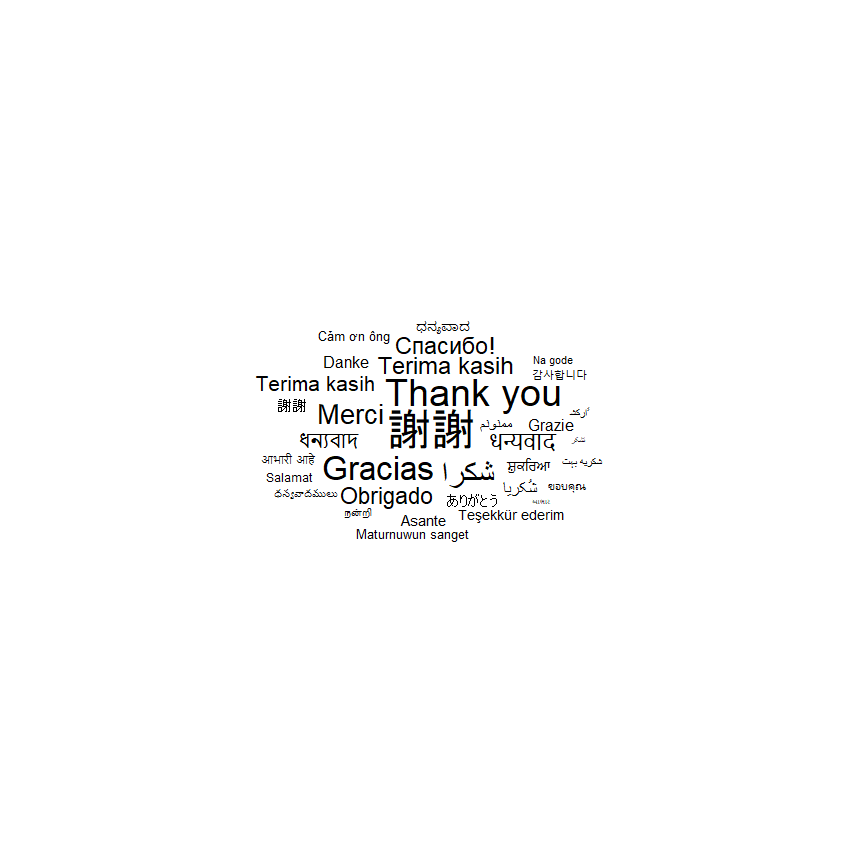Basic word cloud with base R syntax

Alternatively, you could use the `ggwordcloud` and specify the words and frequency (which will determine the relative size of each text) to create the word cloud with a single function. Note that this function provides more arguments which you can customize.

``````# install.packages("ggwordcloud")
library(ggwordcloud)

# Data
df <- thankyou_words_small

set.seed(1)
ggwordcloud(words = df\$word, freq = df\$speakers)``````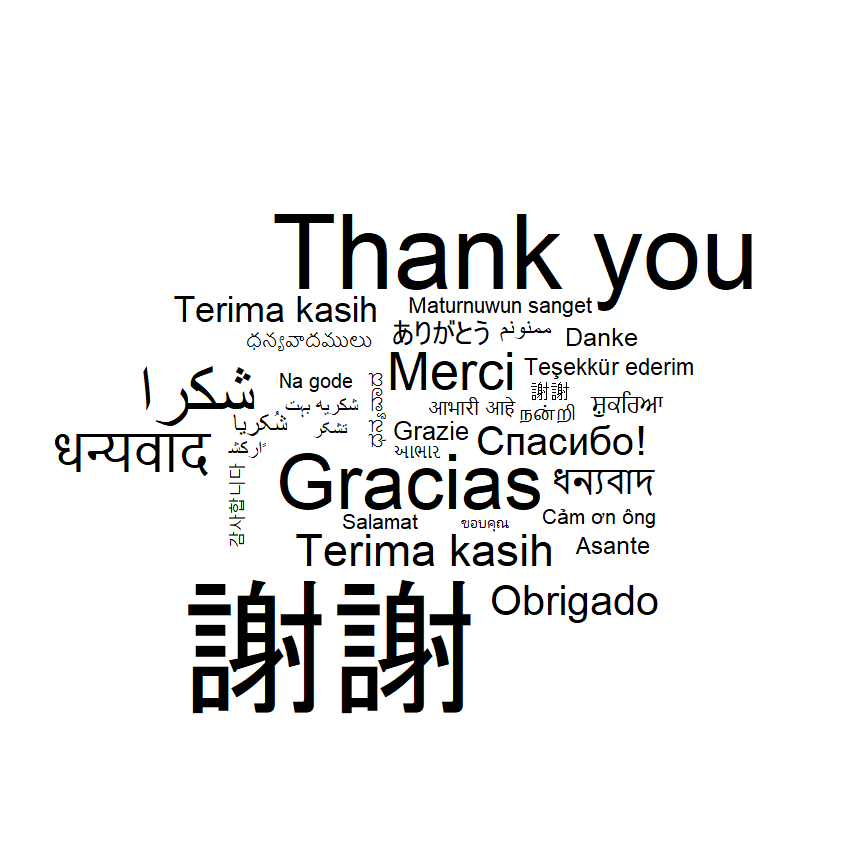Scaling (font size)

The default text scaling of ggplot2 (square root scaling) makes the word cloud look small respect to the plot area. For this reason, you could use the `scale_size_area` function as follows to obtain a better font size control.

``````# install.packages("ggwordcloud")
library(ggwordcloud)

# Data
df <- thankyou_words_small

set.seed(1)
ggplot(df, aes(label = word, size = speakers)) +
geom_text_wordcloud() +
scale_size_area(max_size = 20) +
theme_minimal()``````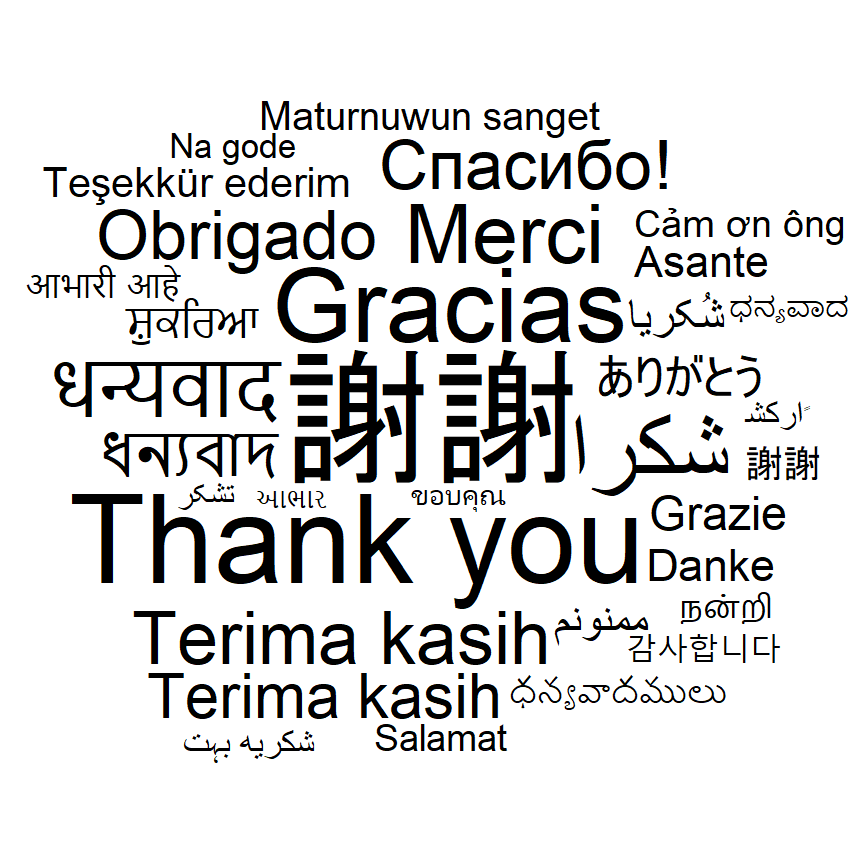Removing texts that not fit

If you have too many words and a big font size you can set the `rm_outside` argument of `geom_text_wordcloud` to `TRUE` or decrease the font size to remove the overflowing texts.

``````# install.packages("ggwordcloud")
library(ggwordcloud)
# install.packages("ggforce")
library(ggforce)

# Data
df <- thankyou_words_small

set.seed(1)
ggplot(df, aes(label = word, size = speakers)) +
geom_text_wordcloud(rm_outside = TRUE) +
scale_size_area(max_size = 60) +
theme_minimal()``````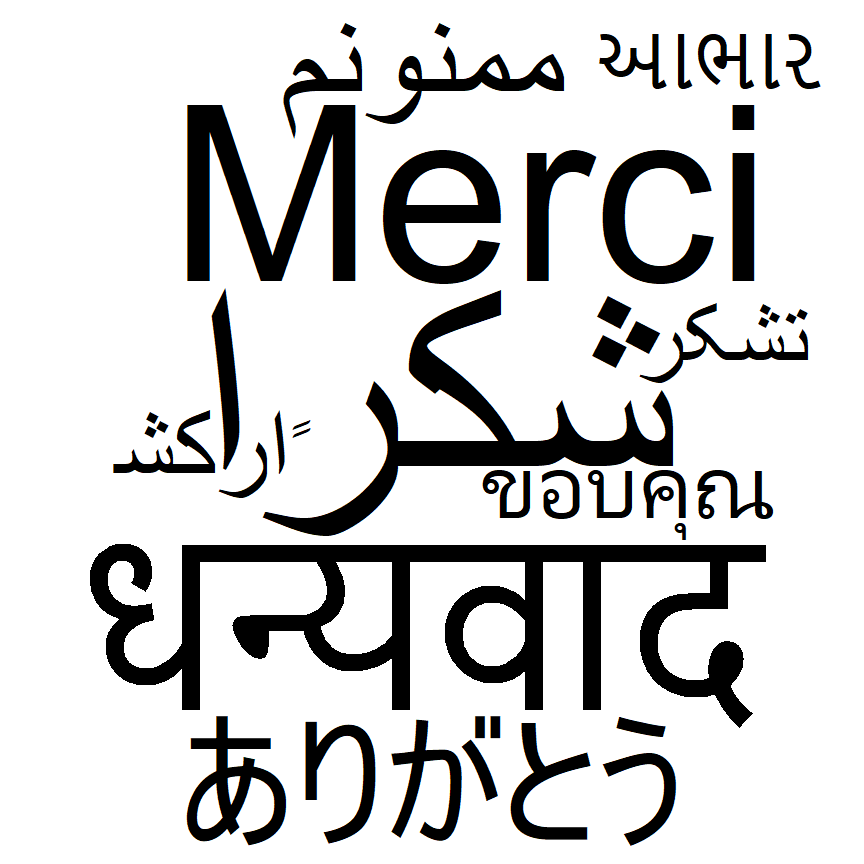Text rotation

Note that you can also rotate the texts with the `angle` argument of `aes`. In the following example we are creating a new column randomly to represent the desired angles to rotate each text.

``````# install.packages("ggwordcloud")
library(ggwordcloud)

set.seed(1)

# Data
df <- thankyou_words_small
df\$angle <- sample(c(0, 45, 60, 90, 120, 180), nrow(df), replace = TRUE)

ggplot(df, aes(label = word, size = speakers, angle = angle)) +
geom_text_wordcloud() +
scale_size_area(max_size = 20) +
theme_minimal()``````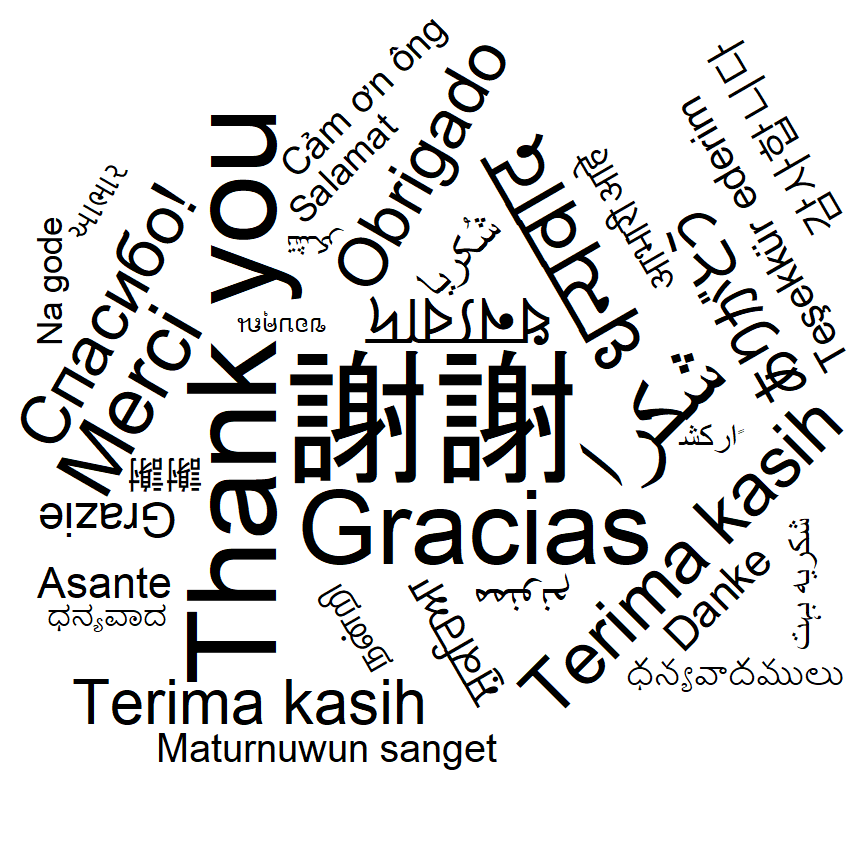## Shape of the word cloud

By default, the shape of the word cloud is circular. However, it is possible to change the shape of the cloud with the `shape` argument of the `geom_text_wordcloud` function. Possible shapes are named `"circle"` (default), `"cardioid"`, `"diamond"`, `"pentagon"`, `"star"`, `"square"`, `"triangle-forward"` and `"triangle-upright"`. In the following blocks of code you can check a couple of examples.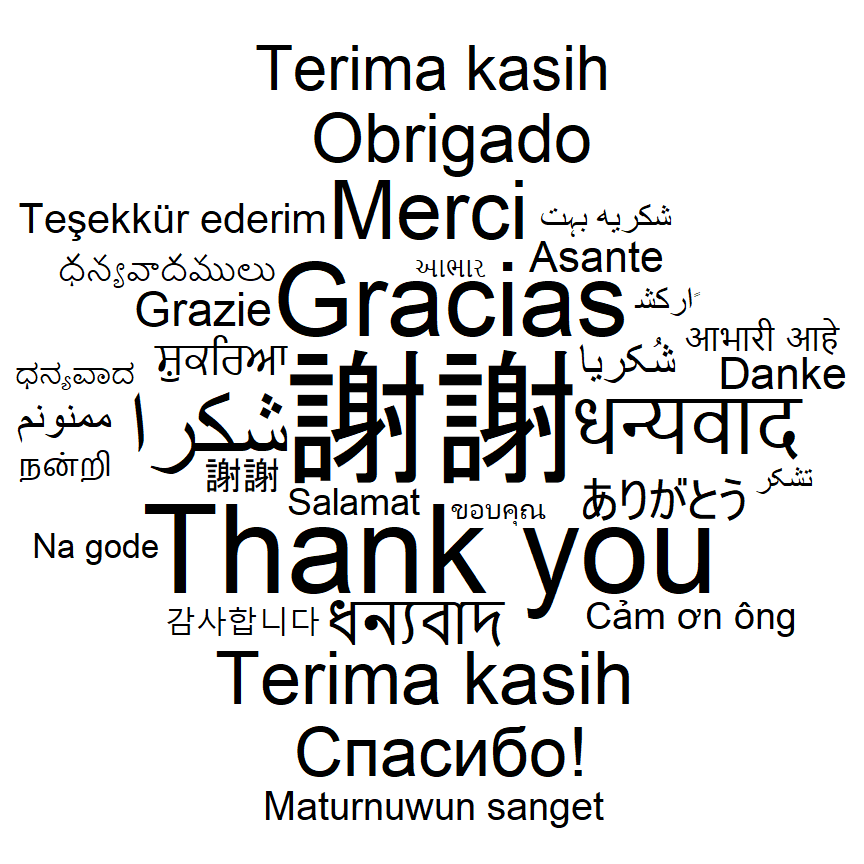Diamond shape

``````# install.packages("ggwordcloud")
library(ggwordcloud)

# Data
df <- thankyou_words_small

set.seed(1)
ggplot(df, aes(label = word, size = speakers)) +
geom_text_wordcloud(shape = "diamond") +
scale_size_area(max_size = 20) +
theme_minimal()``````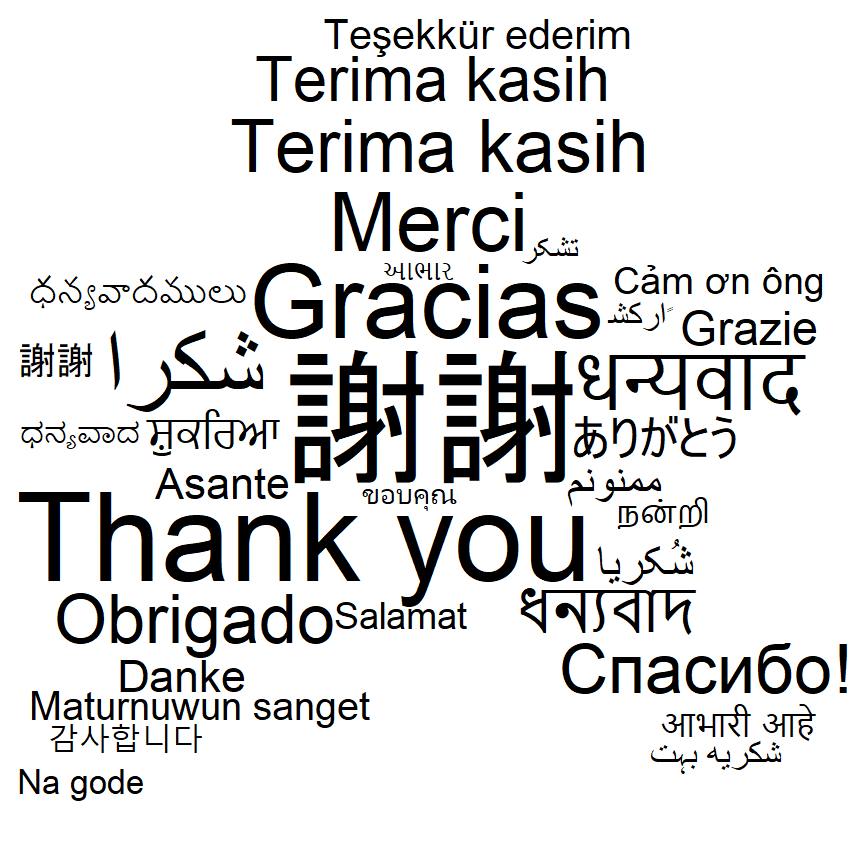Star shape

``````# install.packages("ggwordcloud")
library(ggwordcloud)

# Data
df <- thankyou_words_small

set.seed(1)
ggplot(df, aes(label = word, size = speakers)) +
geom_text_wordcloud(shape = "star") +
scale_size_area(max_size = 20) +
theme_minimal()``````

Alternatively you can use a PNG file to create a mask to place the words within it. Note that the non-transparent pixels of the image will be used as the mask. In the following example we are using a sample PNG file from the `ggwordcloud` package with the shape of a heart to create the mask.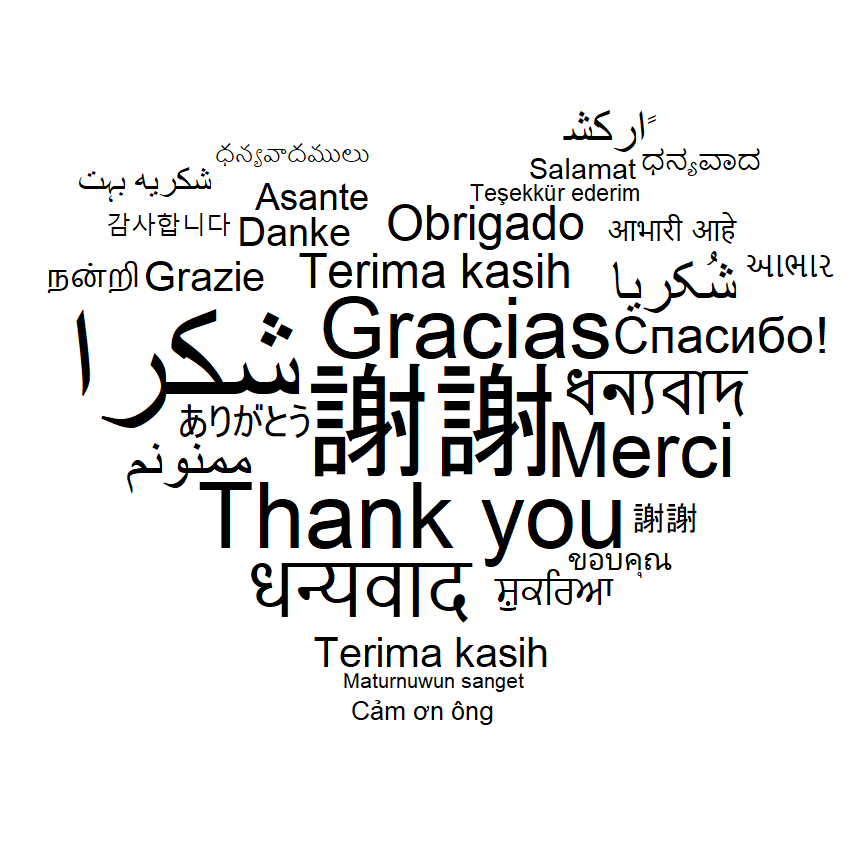``````# install.packages("ggwordcloud")
library(ggwordcloud)

# Data
df <- thankyou_words_small

package = "ggwordcloud", mustWork = TRUE))

set.seed(1)
ggplot(df, aes(label = word, size = speakers)) +
scale_size_area(max_size = 20) +
theme_minimal()``````

## Color of the texts

Unique color

When creating a word cloud with `ggwordcloud` the color of the texts is black by default. Nevertheless, you can customize the color passing a color to the `color` argument of `geom_text_wordcloud`.

``````# install.packages("ggwordcloud")
library(ggwordcloud)

# Data
df <- thankyou_words_small

set.seed(1)
ggplot(df, aes(label = word, size = speakers)) +
geom_text_wordcloud(color = "red") +
scale_size_area(max_size = 20) +
scale_color_discrete("red") +
theme_minimal()``````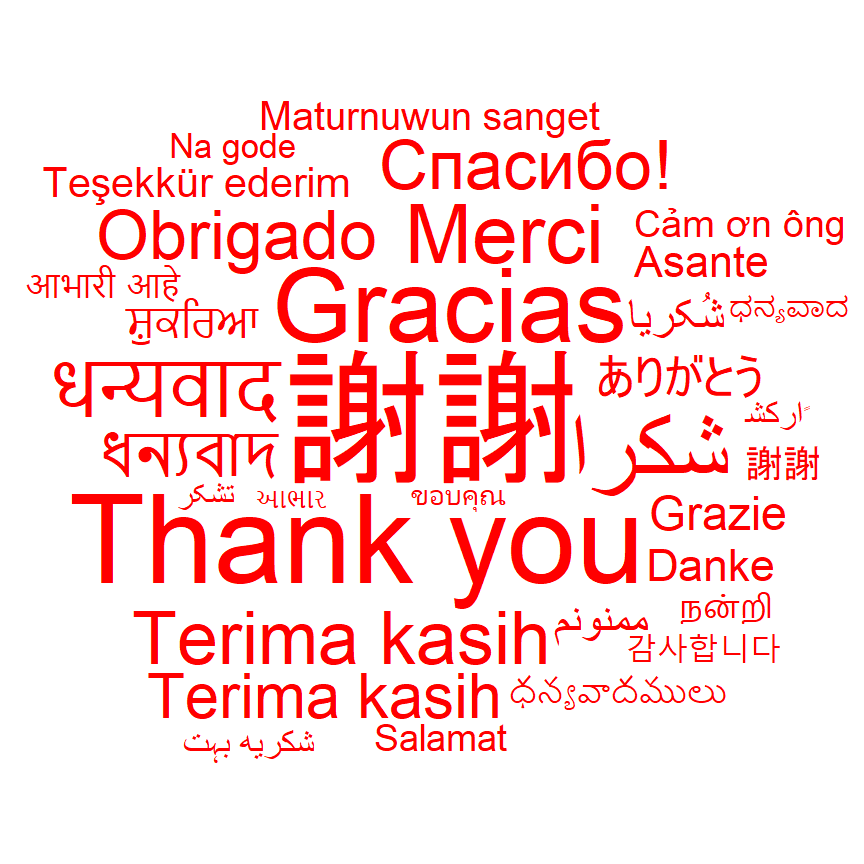Color based on variable

You can also set the color based on a categorical variable. This will allow you to color the text by groups or setting a different color for each text, as in the example below.

``````# install.packages("ggwordcloud")
library(ggwordcloud)

# Data
df <- thankyou_words_small

set.seed(1)
ggplot(df, aes(label = word, size = speakers, color = name)) +
geom_text_wordcloud() +
scale_size_area(max_size = 20) +
theme_minimal()``````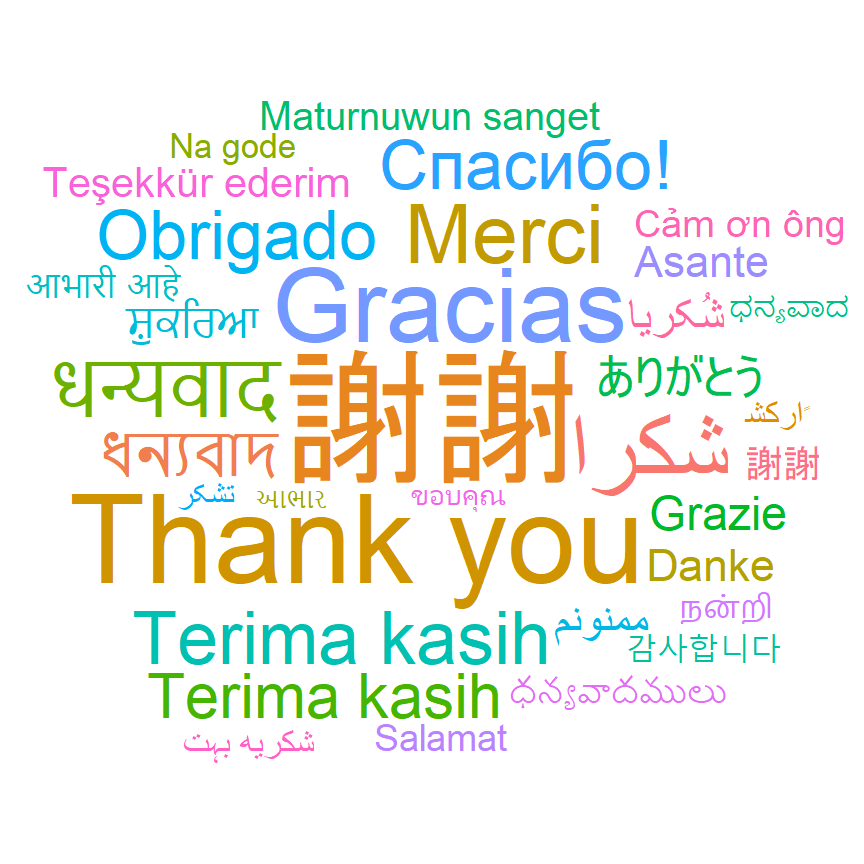Finally, if you want to create a gradient you can pass a numerical variable to the `color` argument of `aes` and use a color scale such as `scale_color_gradient` to create the gradient color scale.

``````# install.packages("ggwordcloud")
library(ggwordcloud)

# Data
df <- thankyou_words_small

set.seed(1)
ggplot(df, aes(label = word, size = speakers, color = speakers)) +
geom_text_wordcloud() +
scale_size_area(max_size = 20) +
theme_minimal() +
scale_color_gradient(low = "darkred", high = "red")``````